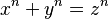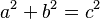# Fermat's Last Theorem facts for kids

Kids Encyclopedia Facts

Fermat's Last Theorem is a very famous idea in mathematics. It says that:

If n is a whole number which is higher than 2 (like 3, 4, 5, 6.....), then the equation$x^n + y^n = z^n$
has no solutions when x, y and z are natural numbers (positive whole numbers (integers) except 0 or 'counting numbers' such as 1, 2, 3....). This means that there are no natural numbers x, y and z for which this equation is true (that is, the values on both sides can never be the same if x, y, z are natural numbers and n is an integer higher than 2).

Pierre de Fermat wrote about it in 1637 inside his copy of a book called Arithmetica. He said "I have a proof of this theorem, but there is not enough space in this margin". However, no correct proof was found for 357 years. It was finally proven in 1995. Mathematicians everywhere think that Fermat, in fact, did not have a good proof of this theorem.

## Relations to other mathematics

Fermat's Last Theorem is a more general form of the equation:$a^2 + b^2 = c^2$. (This comes from the Pythagorean theorem). A special case is when a, b and c are whole numbers. Then they are named a "pythagorean triple". For example: 3, 4 and 5 give 3^2 + 4^2 = 5^2 as 9+16=25, or 5, 12 and 13 give 25+144=169. There are an infinite number of them (they go on forever). Fermat's Last Theorem talks about what happens when the 2 changes to a bigger whole number. It says that then there are no triples when a, b and c are integers greater than or equal to one (meaning that if n is more than two, a, b and c cannot be natural numbers).

## Proof

The proof was made for some values of n (like n=3, n=4, n=5 and n=7). Fermat, Euler, Sophie Germain, and other people did this.

However, the full proof must show that the equation has no solution for all values of n (when n is a whole number bigger than 2). The proof was very difficult to find, and Fermat's Last Theorem needed lots of time to be solved.

An English mathematician named Andrew Wiles found a solution in 1995, 358 years after Fermat wrote about it. Richard Taylor helped him find the solution . The proof took eight years of research. He proved the theorem by first proving the modularity theorem, which was then called the Taniyama–Shimura conjecture. Using Ribet's Theorem, he was able to give a proof for Fermat's Last Theorem. He received the Wolfskehl Prize from Göttingen Academy in June 1997: it amounted to about \$50,000 U.S. dollars.

After a few years of debate, people agreed that Andrew Wiles had solved the problem. Andrew Wiles used a lot of modern mathematics and even created new maths when he made his solution. This mathematics was unknown when Fermat wrote his famous note, so Fermat could not have used it. This leads one to believe that Fermat did not in fact have a complete solution of the problem.

## Images for kidsFermat's Last Theorem Facts for Kids. Kiddle Encyclopedia.